Скачать презентацию Chapter Thirteen Risky Assets Mean of a

2dc6539d991515a51e6eb706e4fe69c9.ppt

• Количество слайдов: 55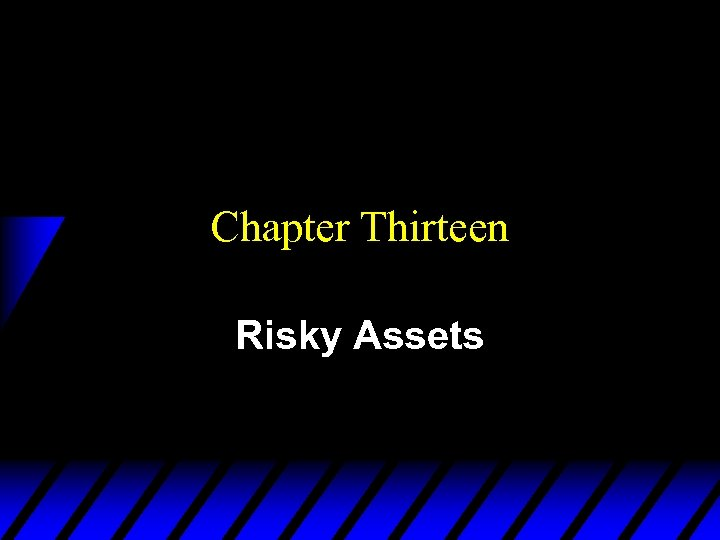Chapter Thirteen Risky Assets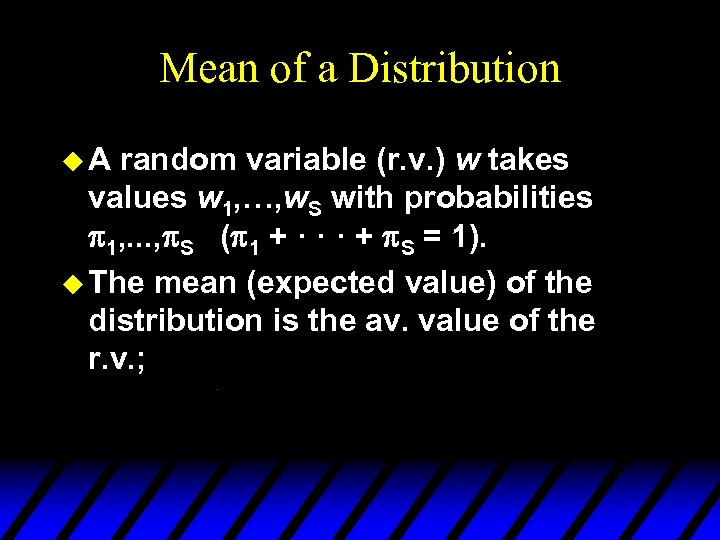Mean of a Distribution u. A random variable (r. v. ) w takes values w 1, …, w. S with probabilities 1, . . . , S ( 1 + · · · + S = 1). u The mean (expected value) of the distribution is the av. value of the r. v. ;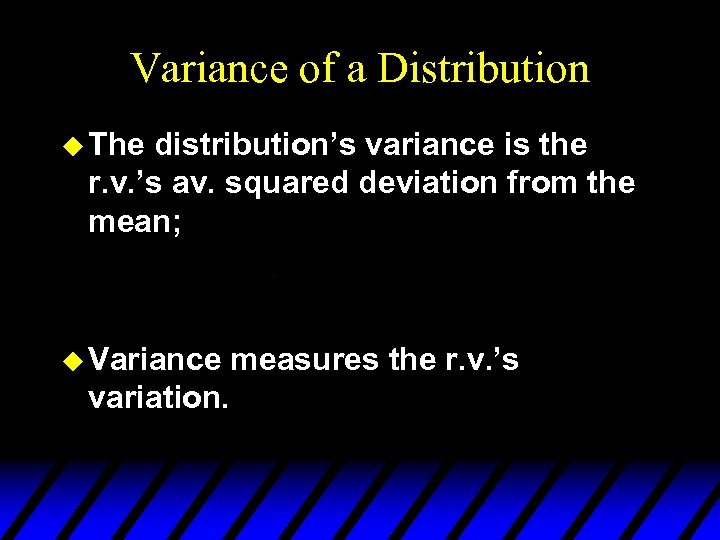Variance of a Distribution u The distribution’s variance is the r. v. ’s av. squared deviation from the mean; u Variance variation. measures the r. v. ’s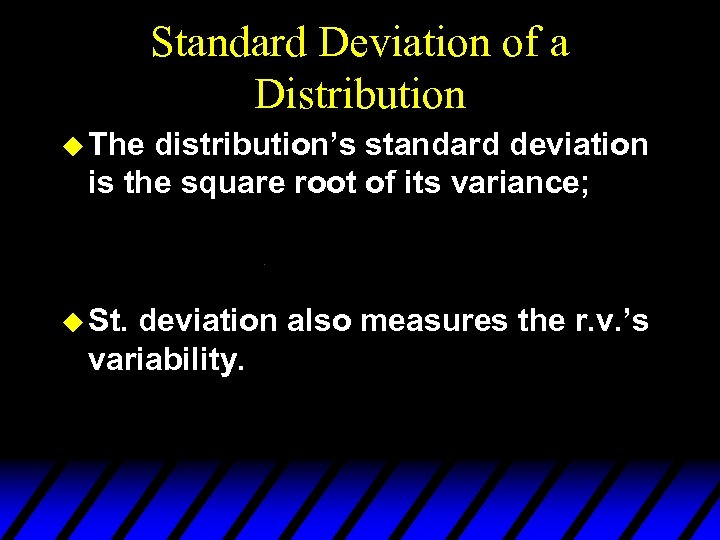Standard Deviation of a Distribution u The distribution’s standard deviation is the square root of its variance; u St. deviation also measures the r. v. ’s variability.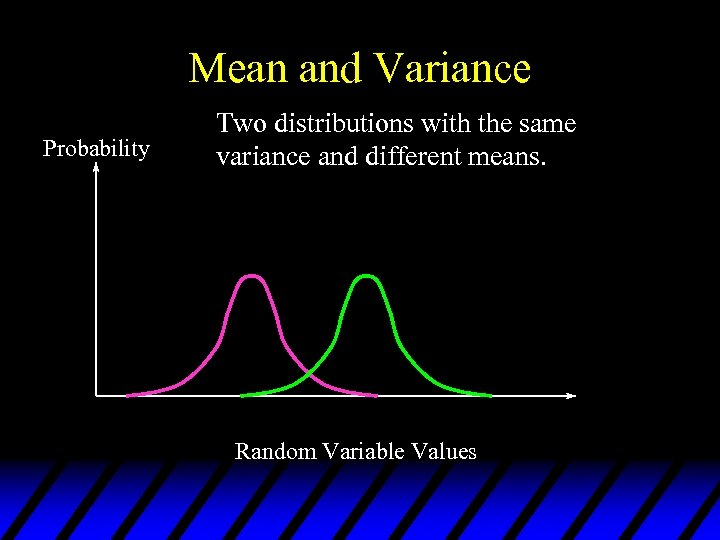Mean and Variance Probability Two distributions with the same variance and different means. Random Variable Values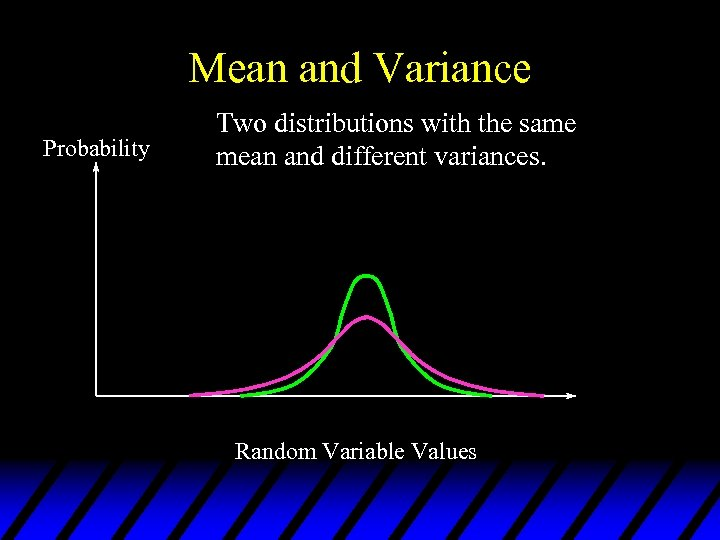Mean and Variance Probability Two distributions with the same mean and different variances. Random Variable Values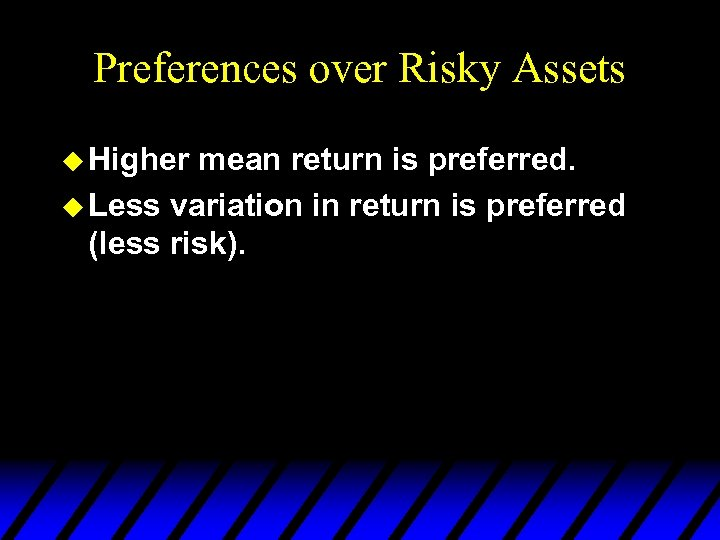Preferences over Risky Assets u Higher mean return is preferred. u Less variation in return is preferred (less risk).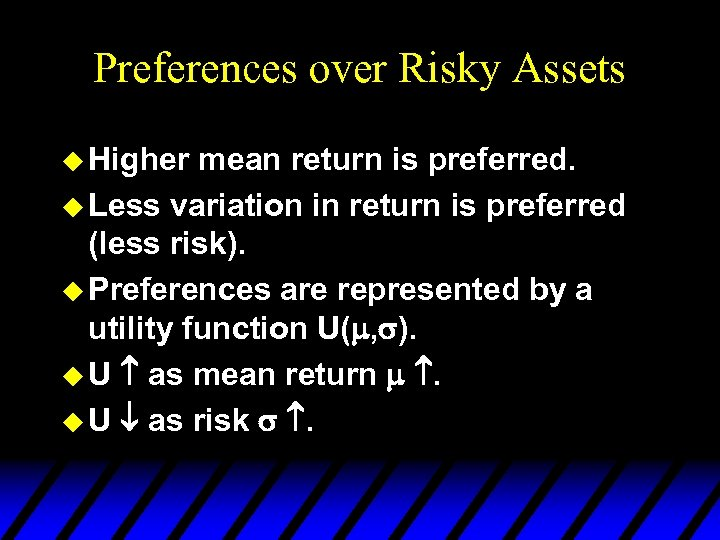Preferences over Risky Assets u Higher mean return is preferred. u Less variation in return is preferred (less risk). u Preferences are represented by a utility function U( , ). u U as mean return . u U as risk .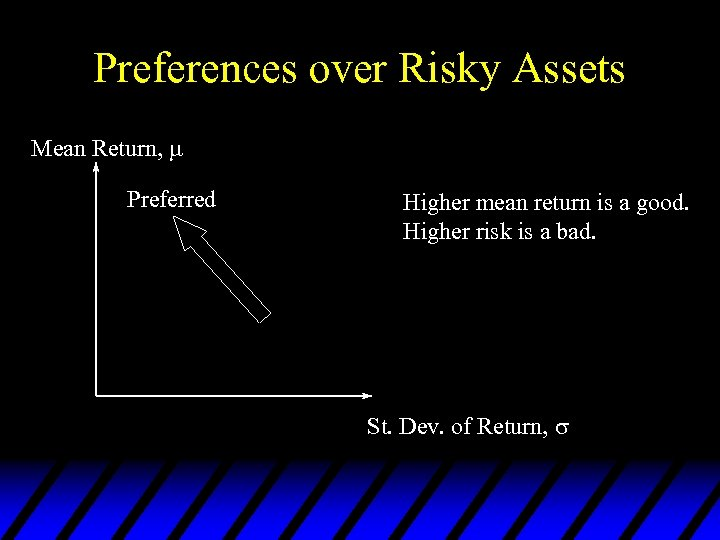Preferences over Risky Assets Mean Return, Preferred Higher mean return is a good. Higher risk is a bad. St. Dev. of Return,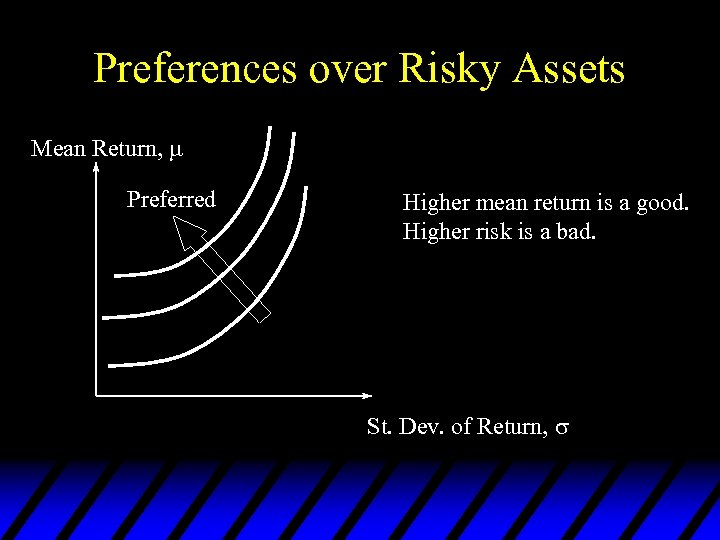Preferences over Risky Assets Mean Return, Preferred Higher mean return is a good. Higher risk is a bad. St. Dev. of Return,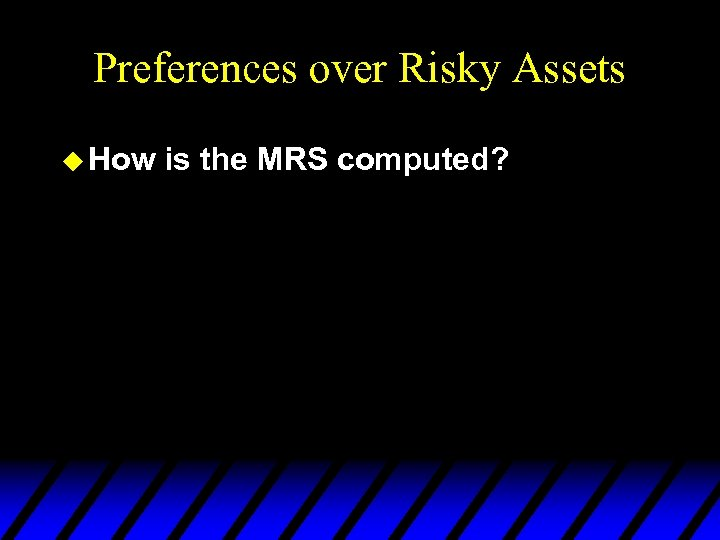Preferences over Risky Assets u How is the MRS computed?Preferences over Risky Assets u How is the MRS computed?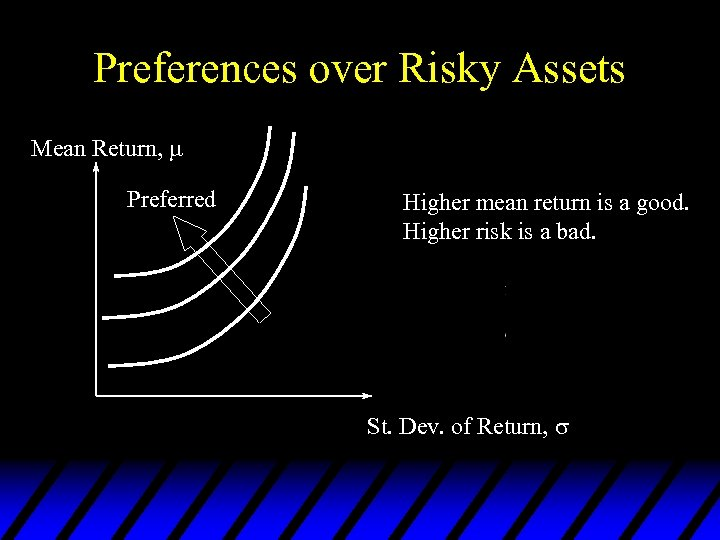Preferences over Risky Assets Mean Return, Preferred Higher mean return is a good. Higher risk is a bad. St. Dev. of Return,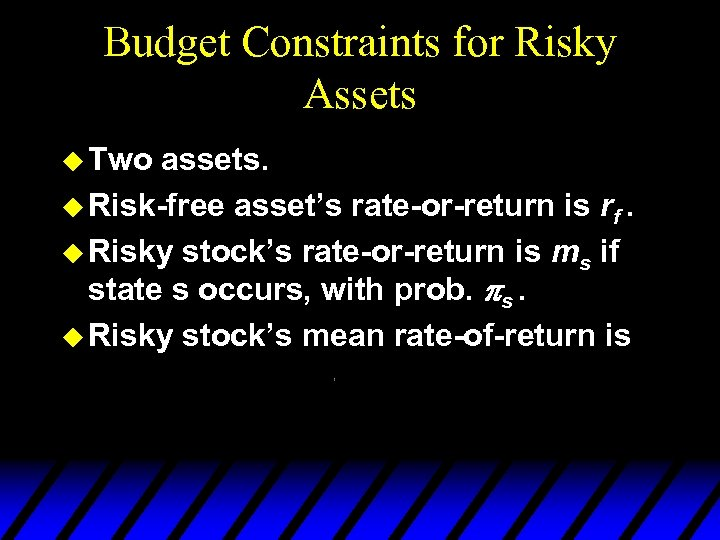Budget Constraints for Risky Assets u Two assets. u Risk-free asset’s rate-or-return is rf. u Risky stock’s rate-or-return is ms if state s occurs, with prob. s. u Risky stock’s mean rate-of-return is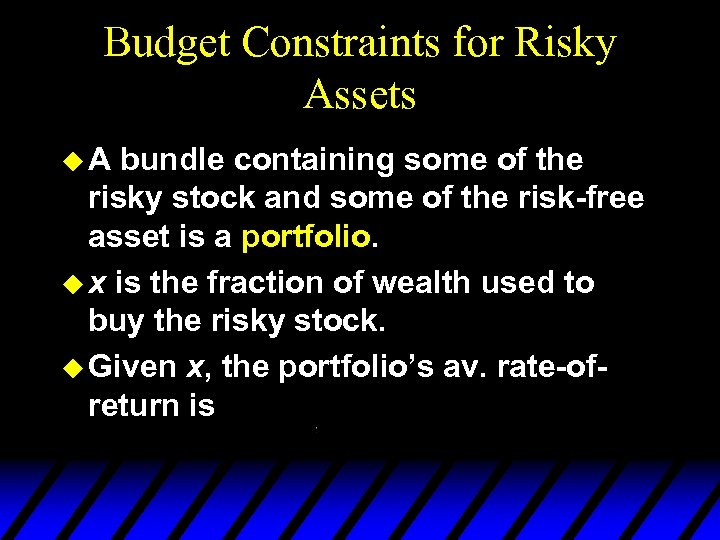Budget Constraints for Risky Assets u. A bundle containing some of the risky stock and some of the risk-free asset is a portfolio. u x is the fraction of wealth used to buy the risky stock. u Given x, the portfolio’s av. rate-ofreturn is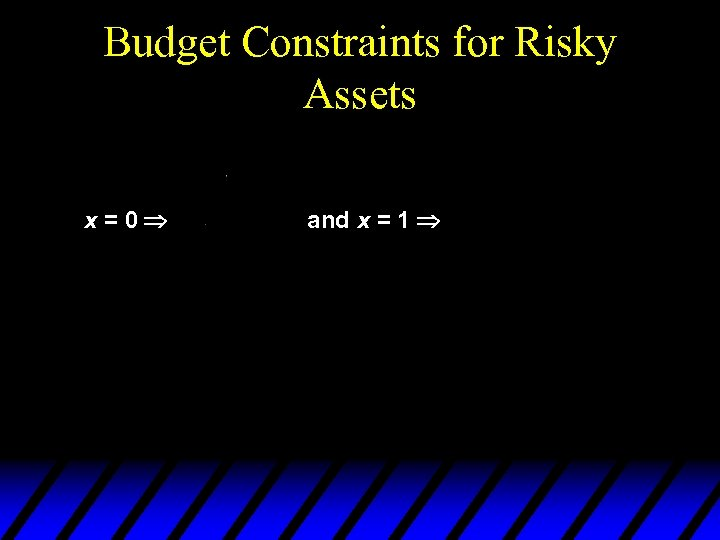Budget Constraints for Risky Assets x=0 and x = 1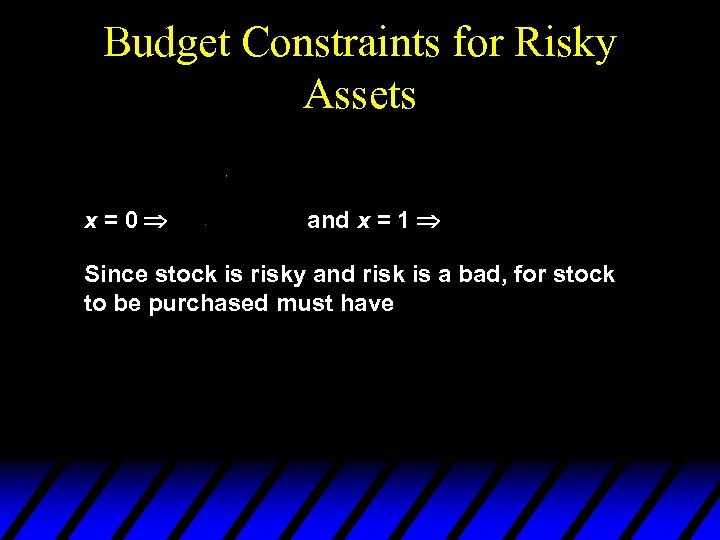Budget Constraints for Risky Assets x=0 and x = 1 Since stock is risky and risk is a bad, for stock to be purchased must have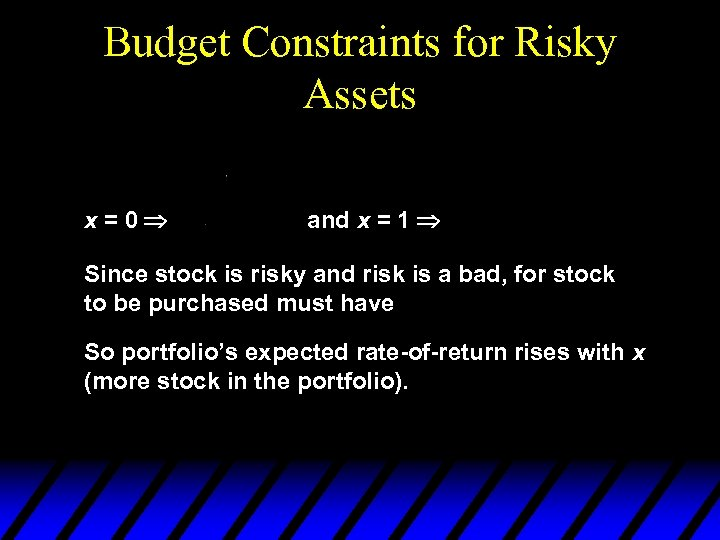Budget Constraints for Risky Assets x=0 and x = 1 Since stock is risky and risk is a bad, for stock to be purchased must have So portfolio’s expected rate-of-return rises with x (more stock in the portfolio).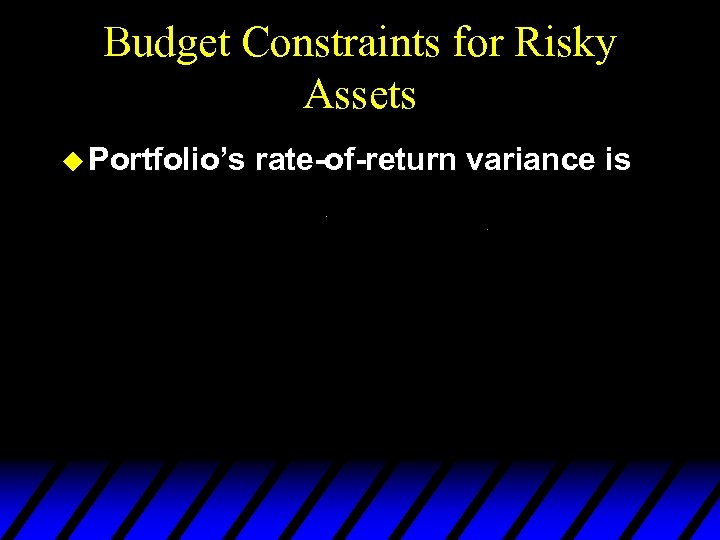Budget Constraints for Risky Assets u Portfolio’s rate-of-return variance is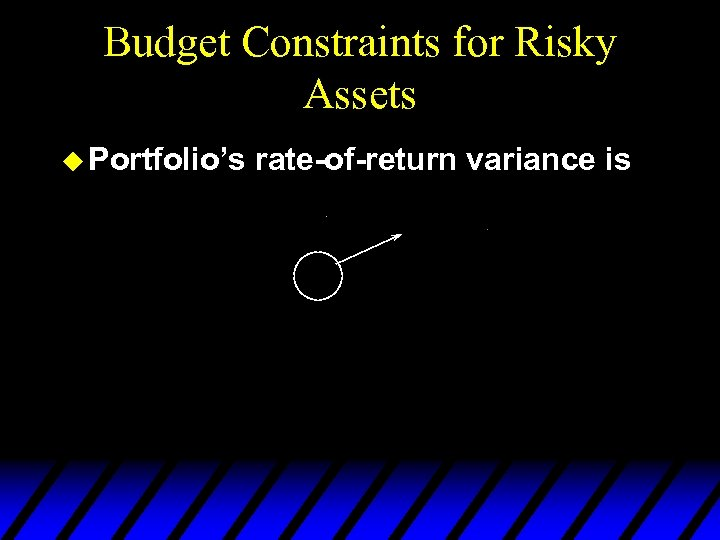Budget Constraints for Risky Assets u Portfolio’s rate-of-return variance is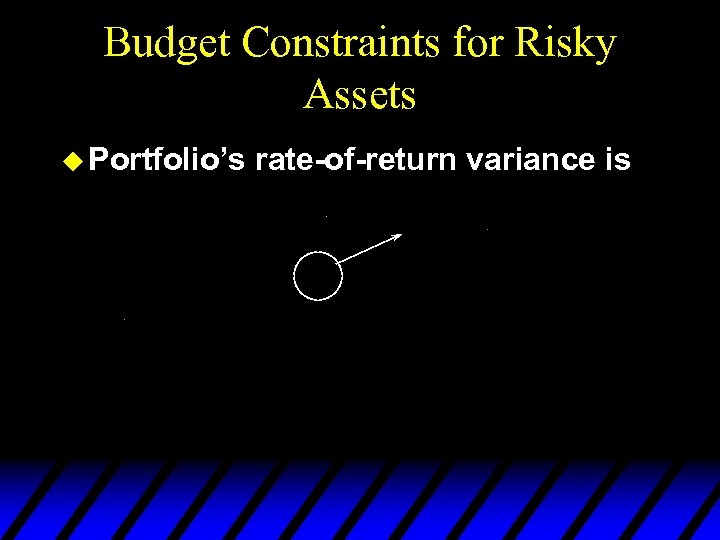Budget Constraints for Risky Assets u Portfolio’s rate-of-return variance is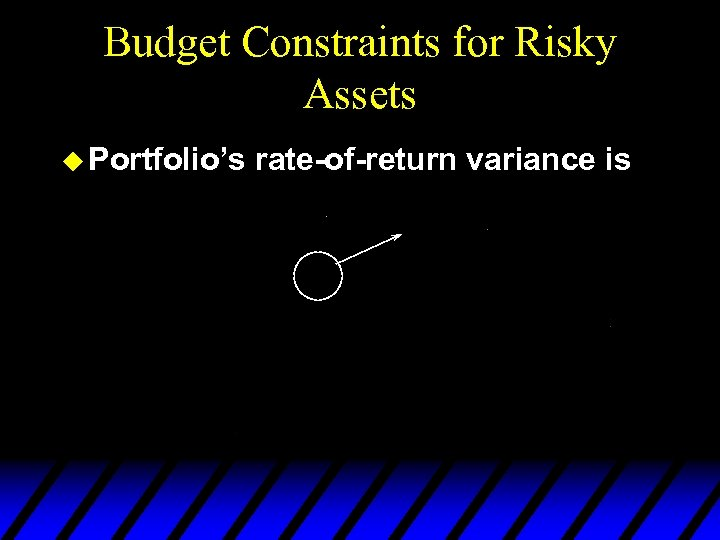Budget Constraints for Risky Assets u Portfolio’s rate-of-return variance is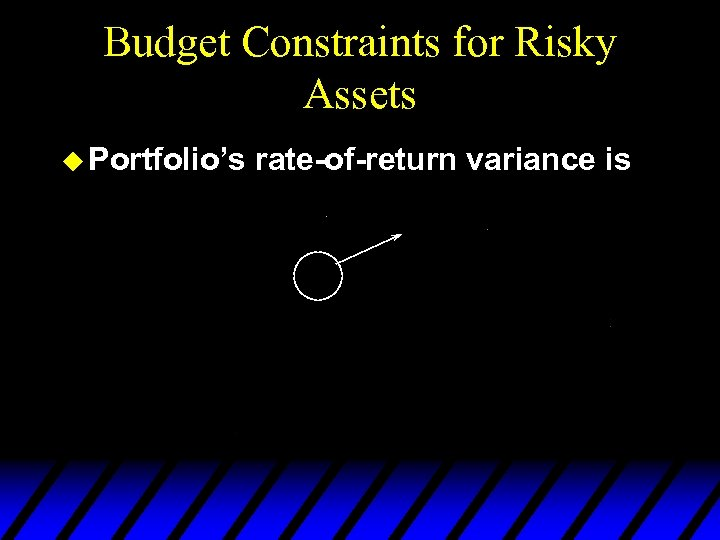Budget Constraints for Risky Assets u Portfolio’s rate-of-return variance is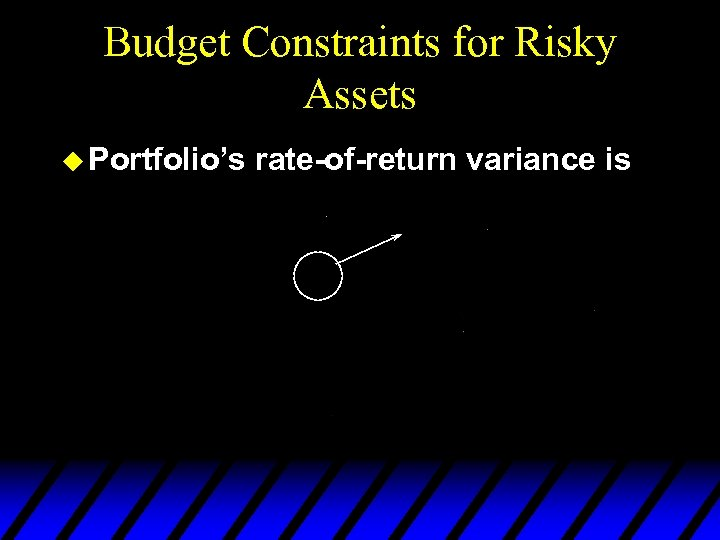Budget Constraints for Risky Assets u Portfolio’s rate-of-return variance is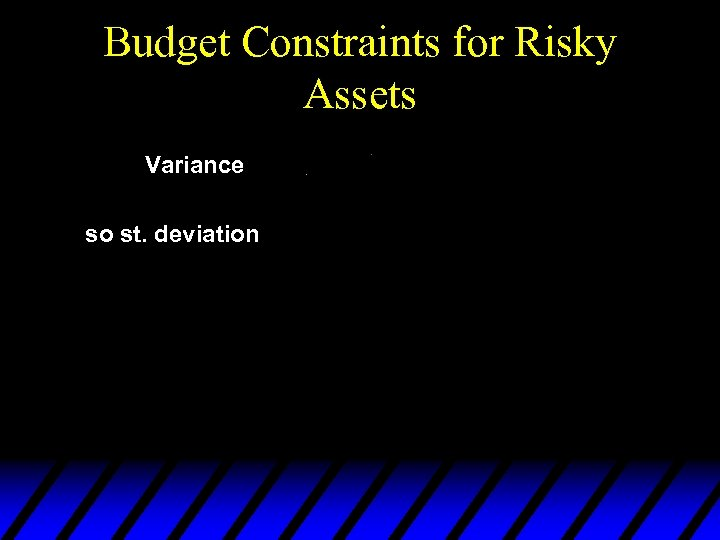Budget Constraints for Risky Assets Variance so st. deviation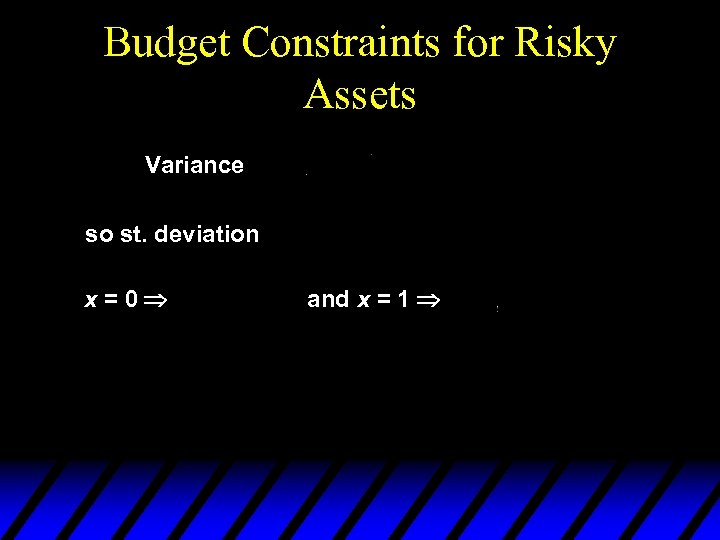Budget Constraints for Risky Assets Variance so st. deviation x=0 and x = 1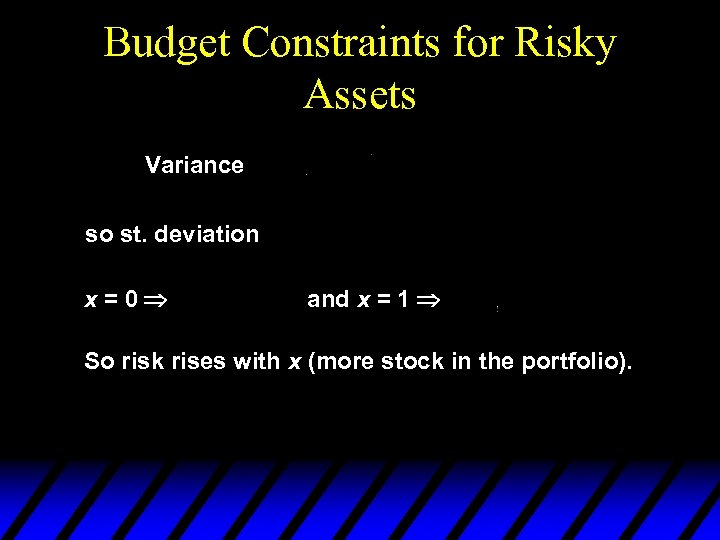Budget Constraints for Risky Assets Variance so st. deviation x=0 and x = 1 So risk rises with x (more stock in the portfolio).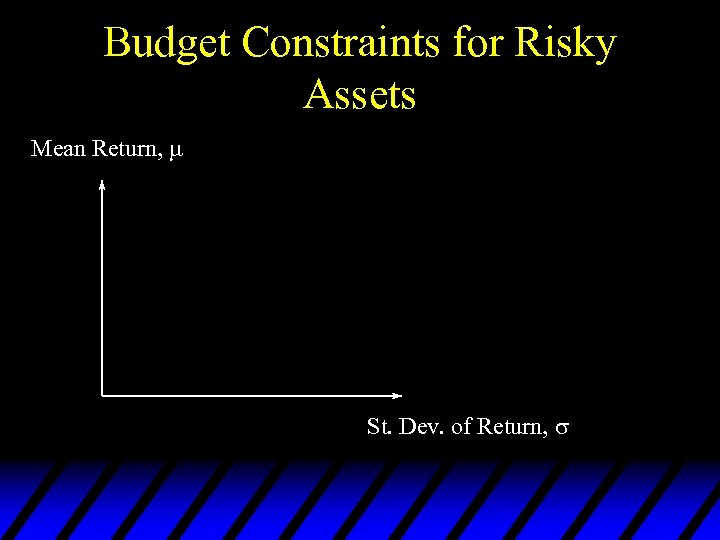Budget Constraints for Risky Assets Mean Return, St. Dev. of Return,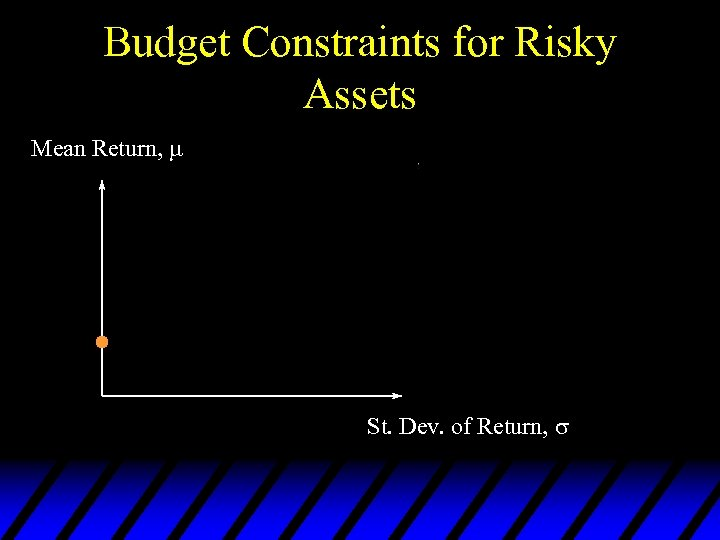Budget Constraints for Risky Assets Mean Return, St. Dev. of Return,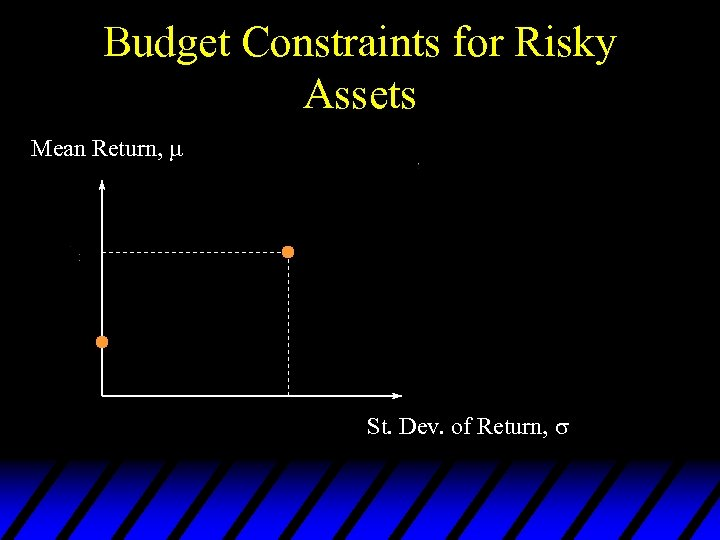Budget Constraints for Risky Assets Mean Return, St. Dev. of Return,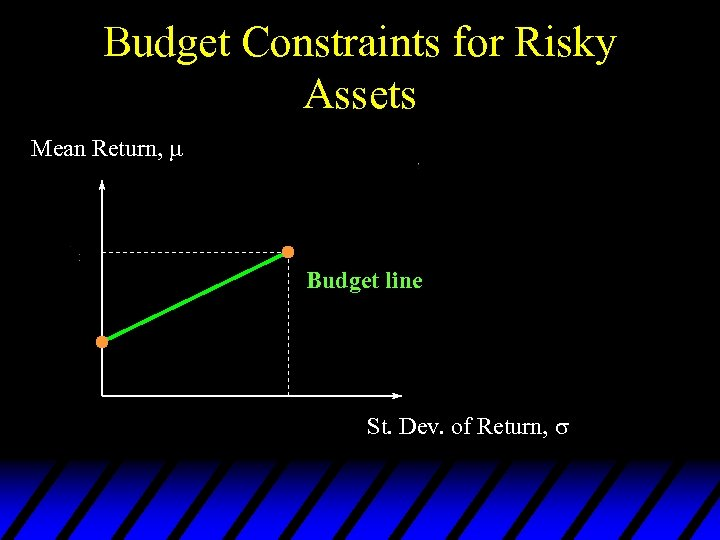Budget Constraints for Risky Assets Mean Return, Budget line St. Dev. of Return,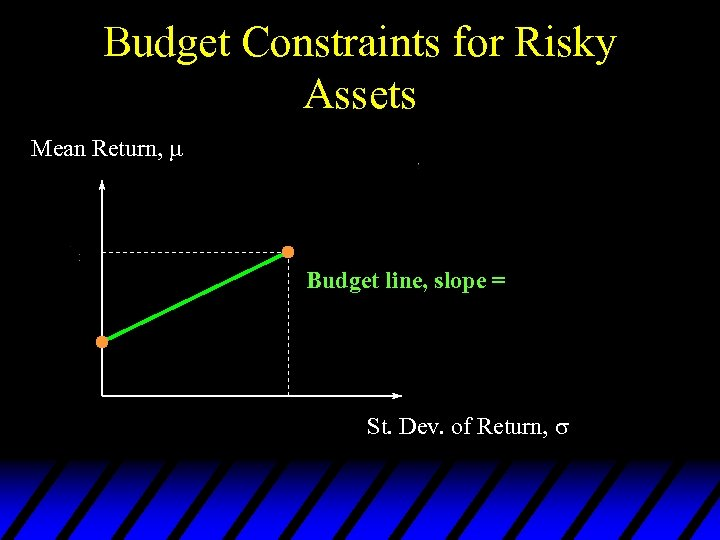Budget Constraints for Risky Assets Mean Return, Budget line, slope = St. Dev. of Return,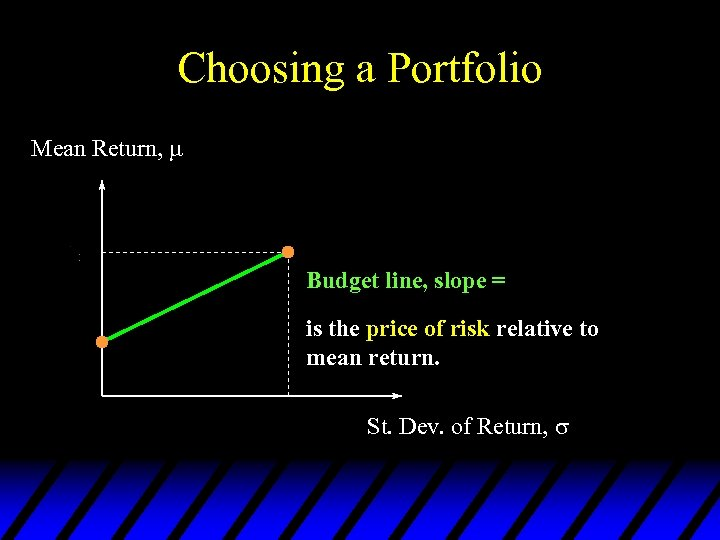Choosing a Portfolio Mean Return, Budget line, slope = is the price of risk relative to mean return. St. Dev. of Return,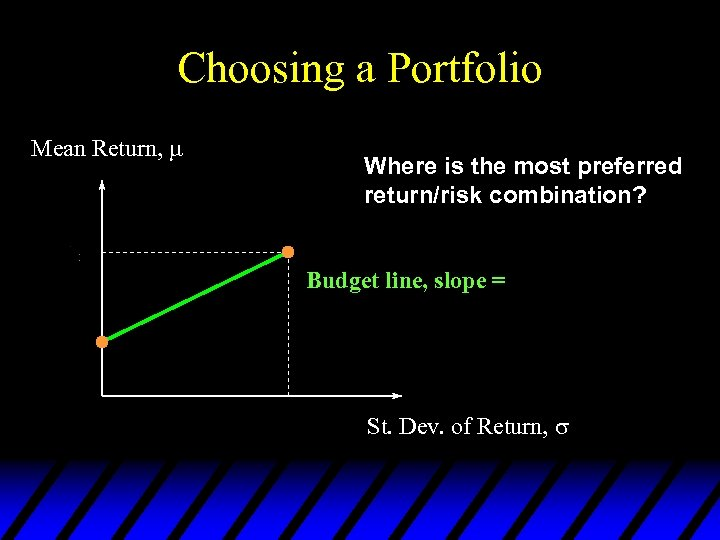Choosing a Portfolio Mean Return, Where is the most preferred return/risk combination? Budget line, slope = St. Dev. of Return,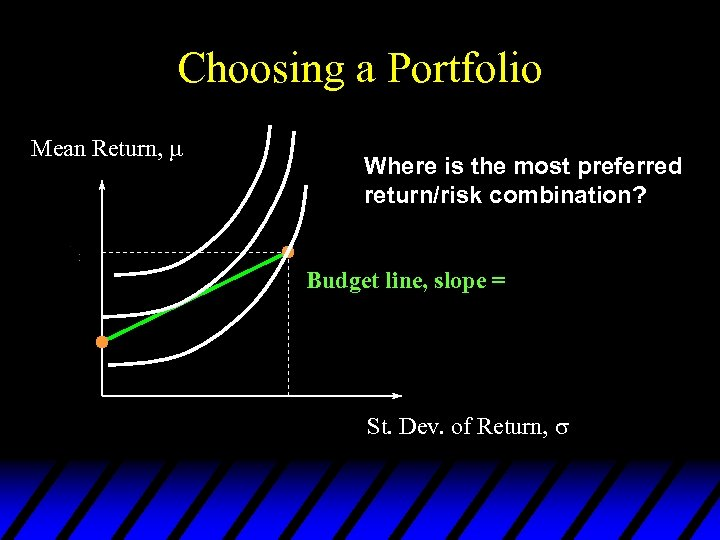Choosing a Portfolio Mean Return, Where is the most preferred return/risk combination? Budget line, slope = St. Dev. of Return,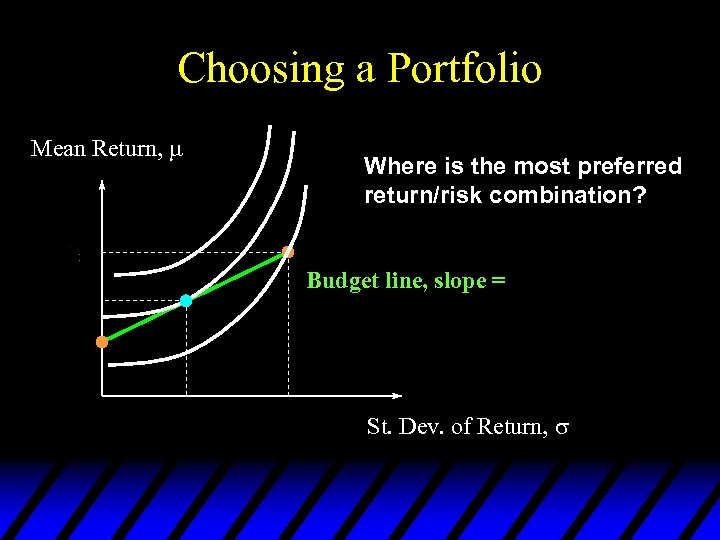Choosing a Portfolio Mean Return, Where is the most preferred return/risk combination? Budget line, slope = St. Dev. of Return,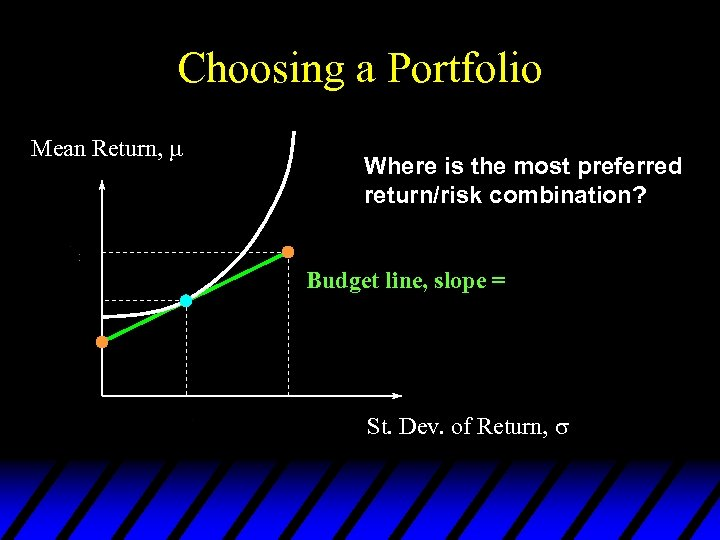Choosing a Portfolio Mean Return, Where is the most preferred return/risk combination? Budget line, slope = St. Dev. of Return,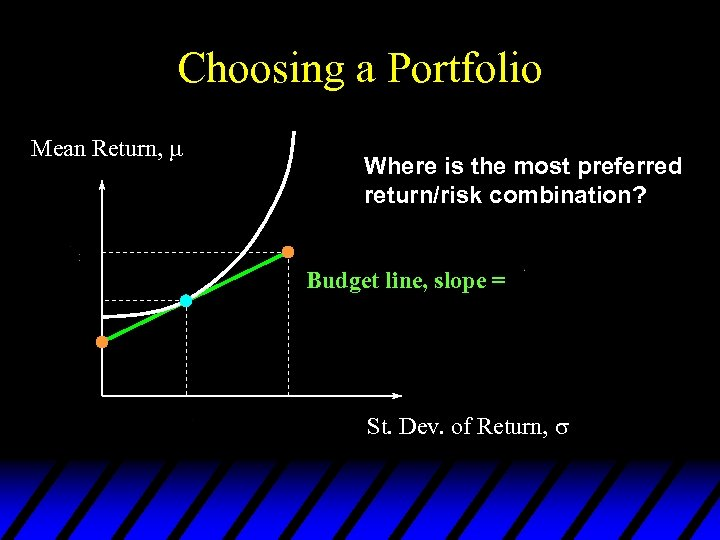Choosing a Portfolio Mean Return, Where is the most preferred return/risk combination? Budget line, slope = St. Dev. of Return,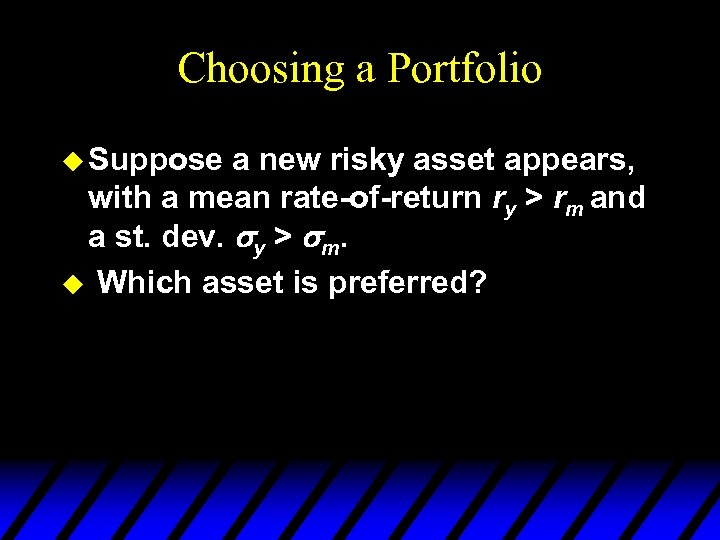Choosing a Portfolio u Suppose a new risky asset appears, with a mean rate-of-return ry > rm and a st. dev. y > m. u Which asset is preferred?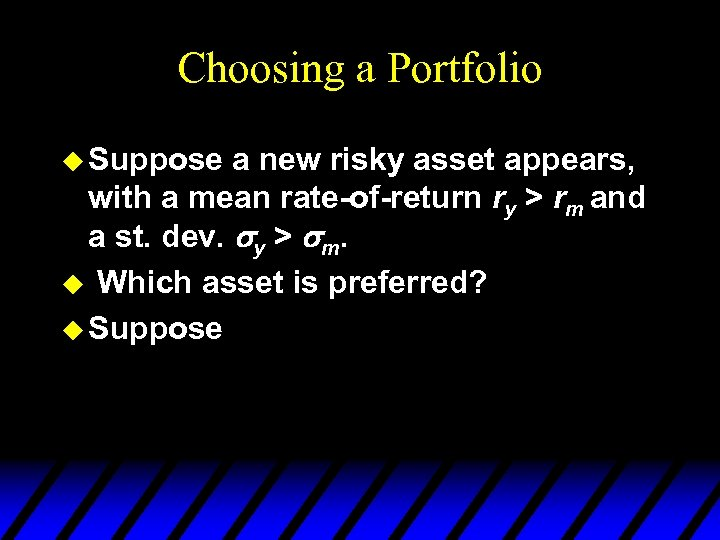Choosing a Portfolio u Suppose a new risky asset appears, with a mean rate-of-return ry > rm and a st. dev. y > m. u Which asset is preferred? u Suppose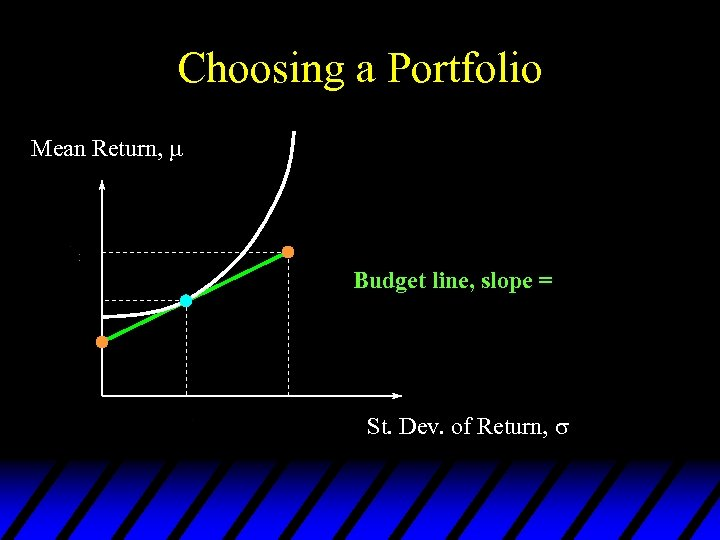Choosing a Portfolio Mean Return, Budget line, slope = St. Dev. of Return,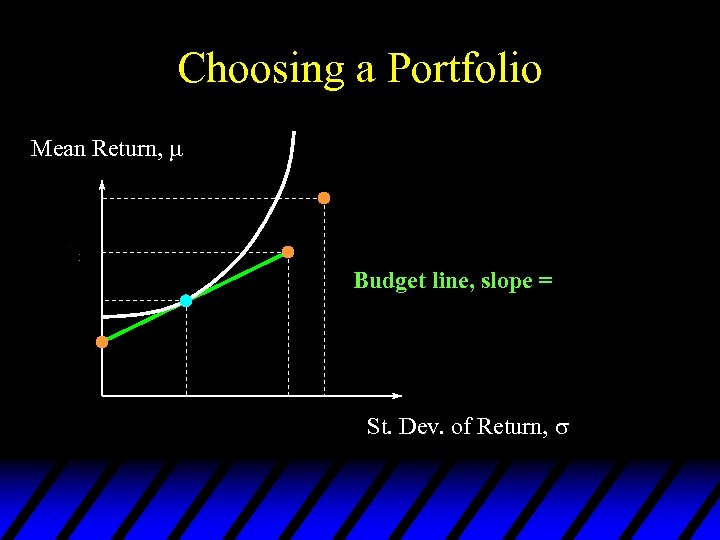Choosing a Portfolio Mean Return, Budget line, slope = St. Dev. of Return,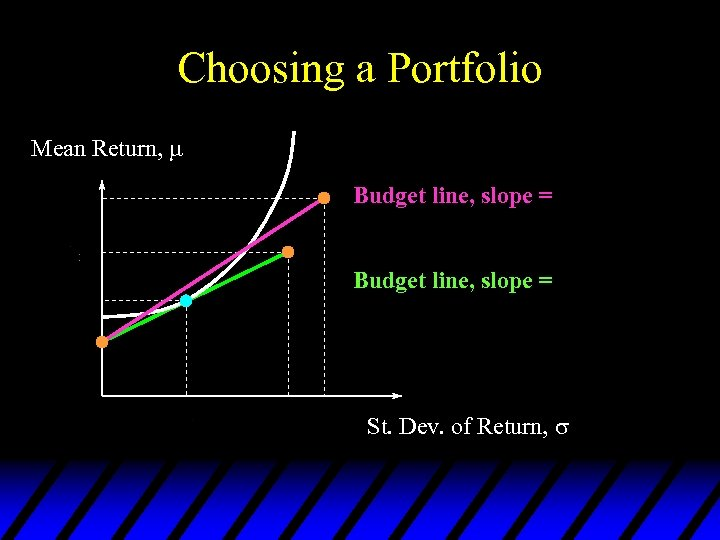Choosing a Portfolio Mean Return, Budget line, slope = St. Dev. of Return,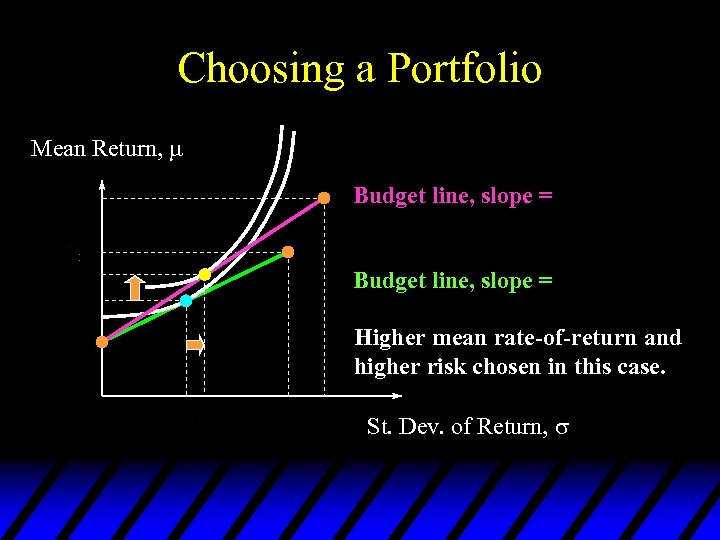Choosing a Portfolio Mean Return, Budget line, slope = Higher mean rate-of-return and higher risk chosen in this case. St. Dev. of Return,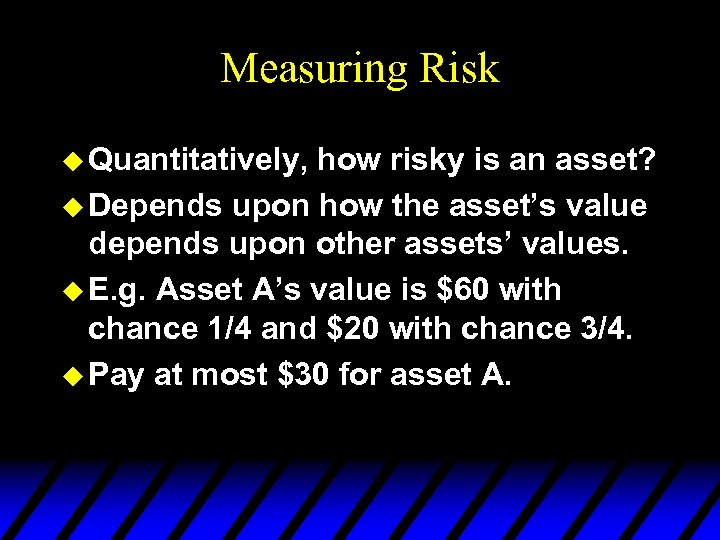Measuring Risk u Quantitatively, how risky is an asset? u Depends upon how the asset’s value depends upon other assets’ values. u E. g. Asset A’s value is \$60 with chance 1/4 and \$20 with chance 3/4. u Pay at most \$30 for asset A.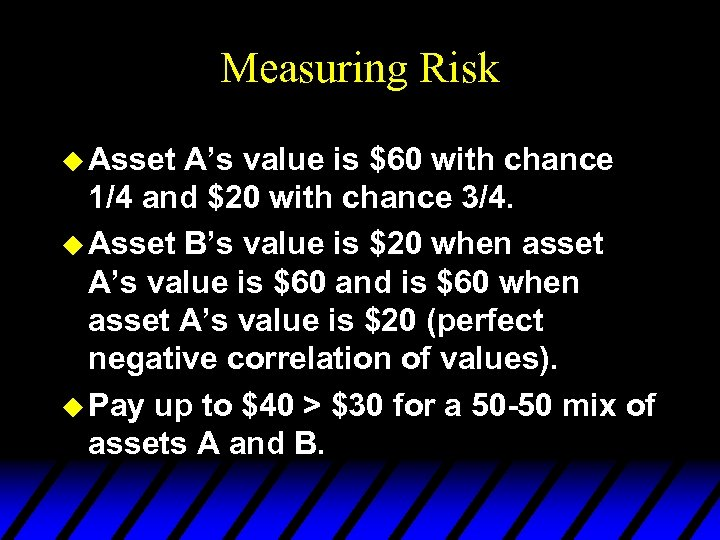Measuring Risk u Asset A’s value is \$60 with chance 1/4 and \$20 with chance 3/4. u Asset B’s value is \$20 when asset A’s value is \$60 and is \$60 when asset A’s value is \$20 (perfect negative correlation of values). u Pay up to \$40 > \$30 for a 50 -50 mix of assets A and B.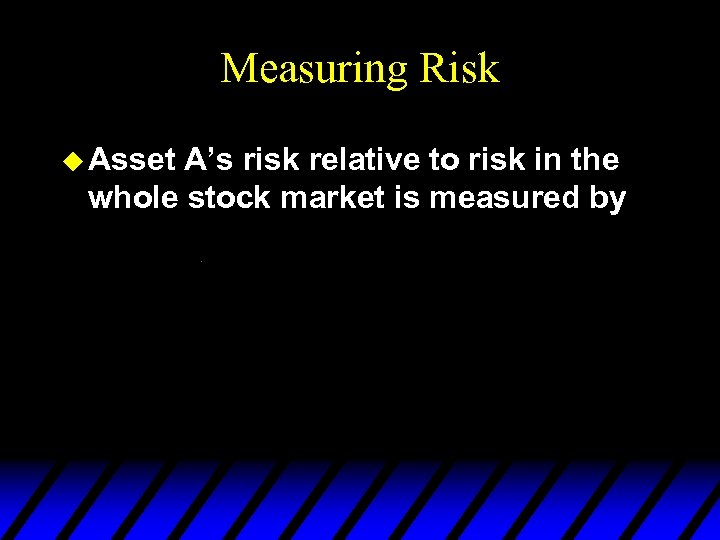Measuring Risk u Asset A’s risk relative to risk in the whole stock market is measured by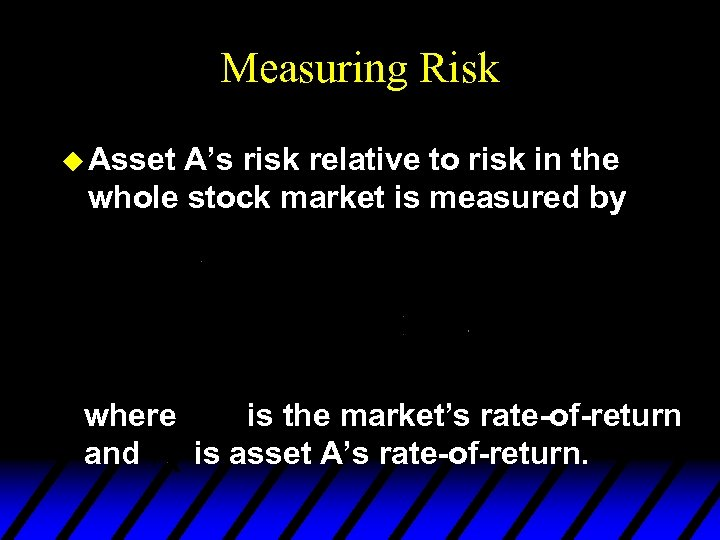Measuring Risk u Asset A’s risk relative to risk in the whole stock market is measured by where is the market’s rate-of-return and is asset A’s rate-of-return.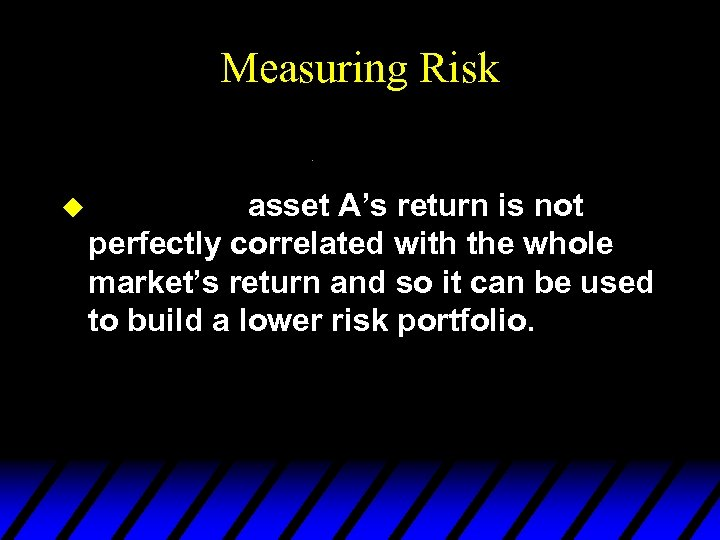Measuring Risk u asset A’s return is not perfectly correlated with the whole market’s return and so it can be used to build a lower risk portfolio.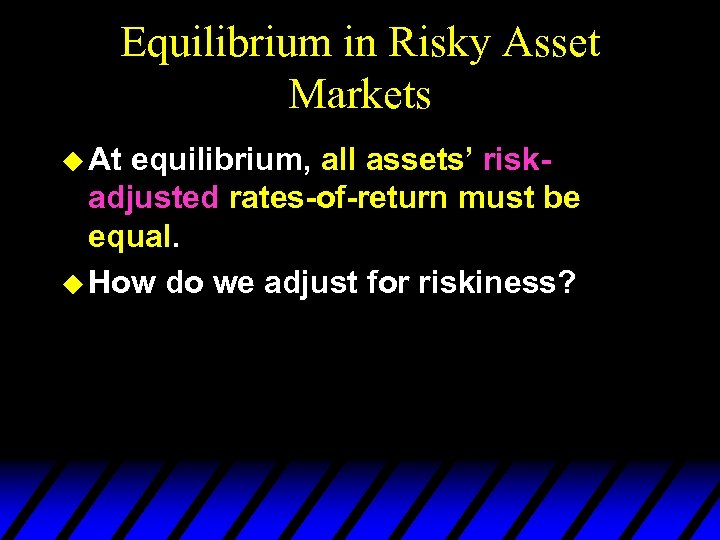Equilibrium in Risky Asset Markets u At equilibrium, all assets’ riskadjusted rates-of-return must be equal. u How do we adjust for riskiness?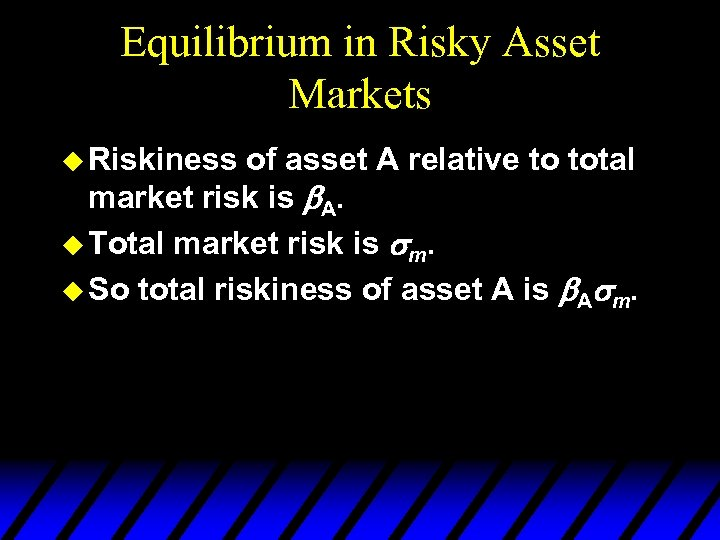Equilibrium in Risky Asset Markets u Riskiness of asset A relative to total market risk is A. u Total market risk is m. u So total riskiness of asset A is A m.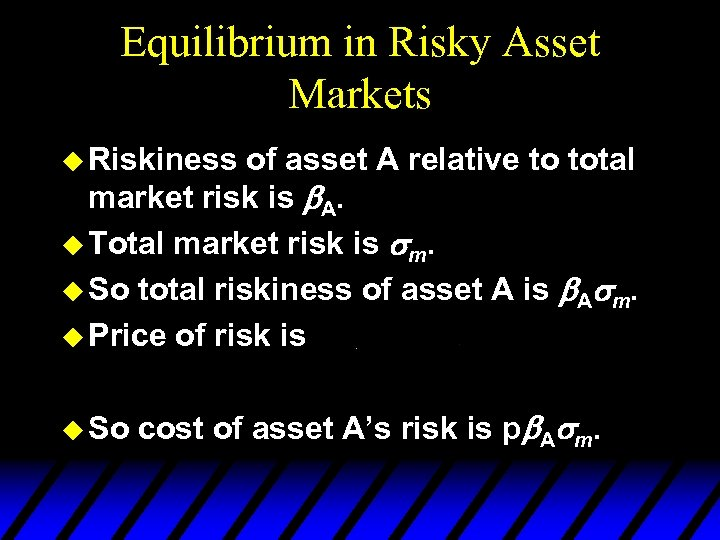Equilibrium in Risky Asset Markets u Riskiness of asset A relative to total market risk is A. u Total market risk is m. u So total riskiness of asset A is A m. u Price of risk is u So cost of asset A’s risk is p A m.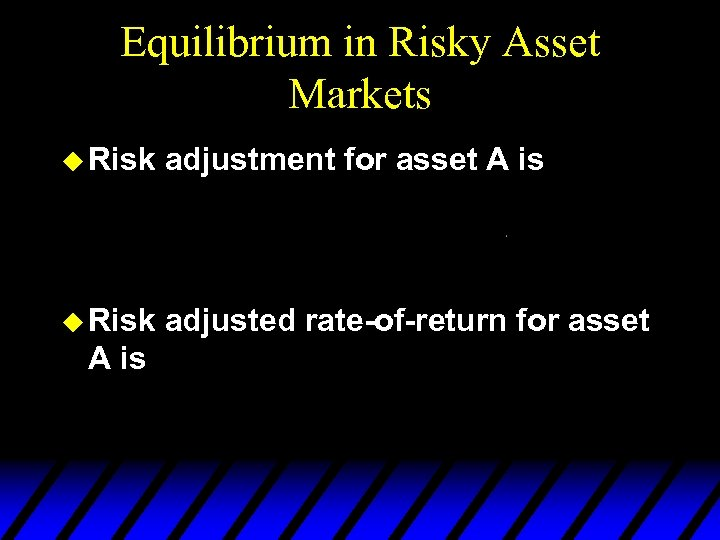Equilibrium in Risky Asset Markets u Risk adjustment for asset A is u Risk adjusted rate-of-return for asset A is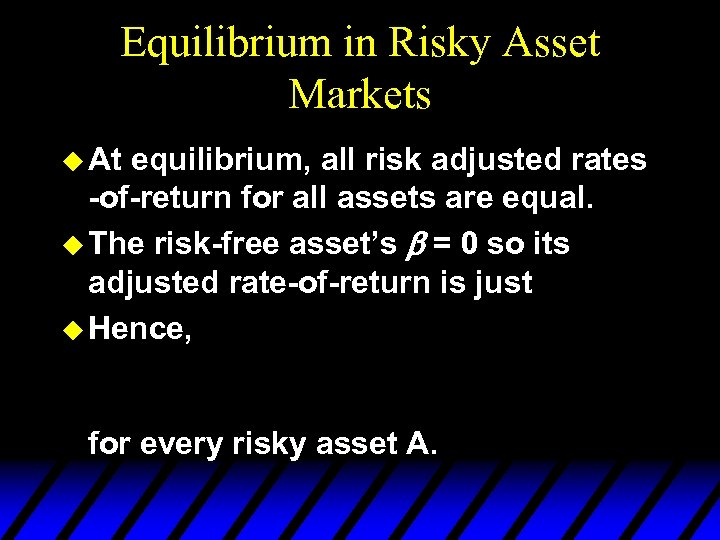Equilibrium in Risky Asset Markets u At equilibrium, all risk adjusted rates -of-return for all assets are equal. u The risk-free asset’s = 0 so its adjusted rate-of-return is just u Hence, for every risky asset A.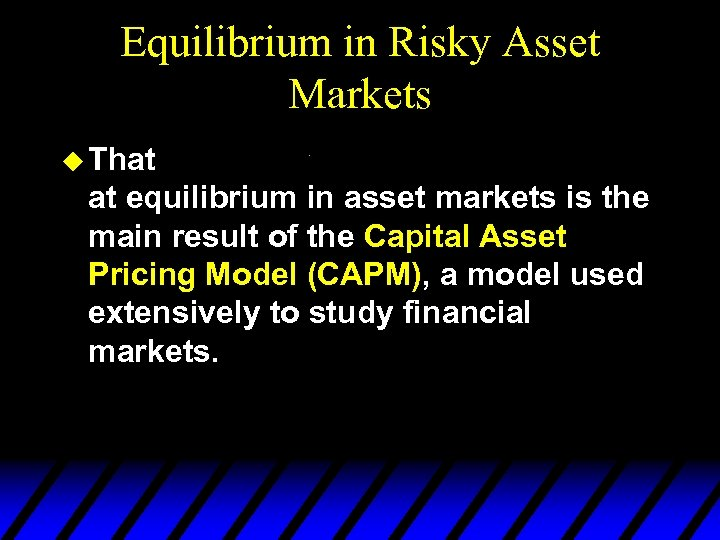Equilibrium in Risky Asset Markets u That at equilibrium in asset markets is the main result of the Capital Asset Pricing Model (CAPM), a model used extensively to study financial markets.